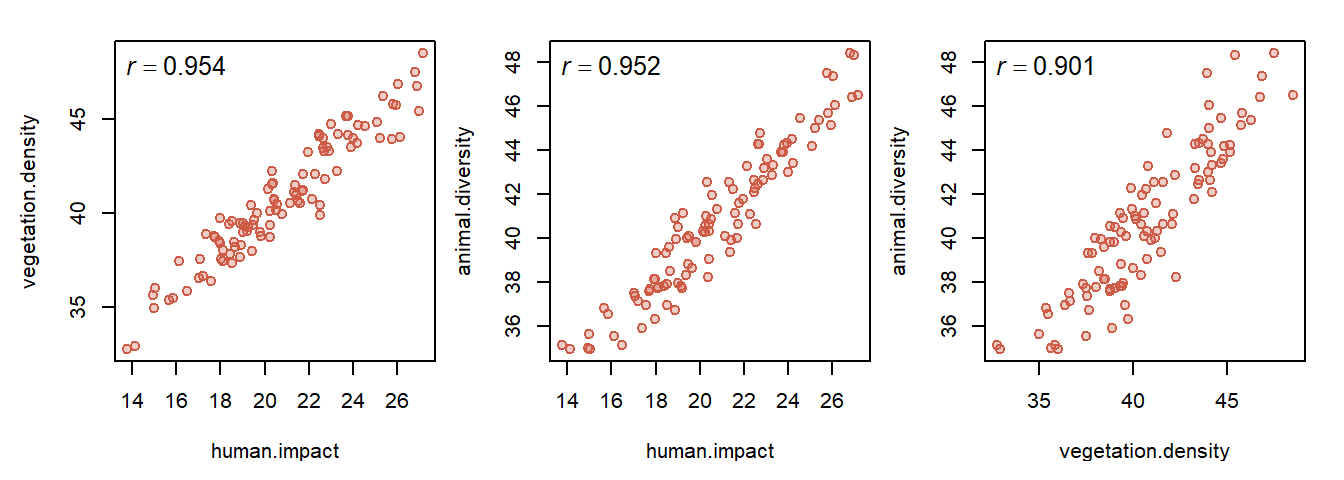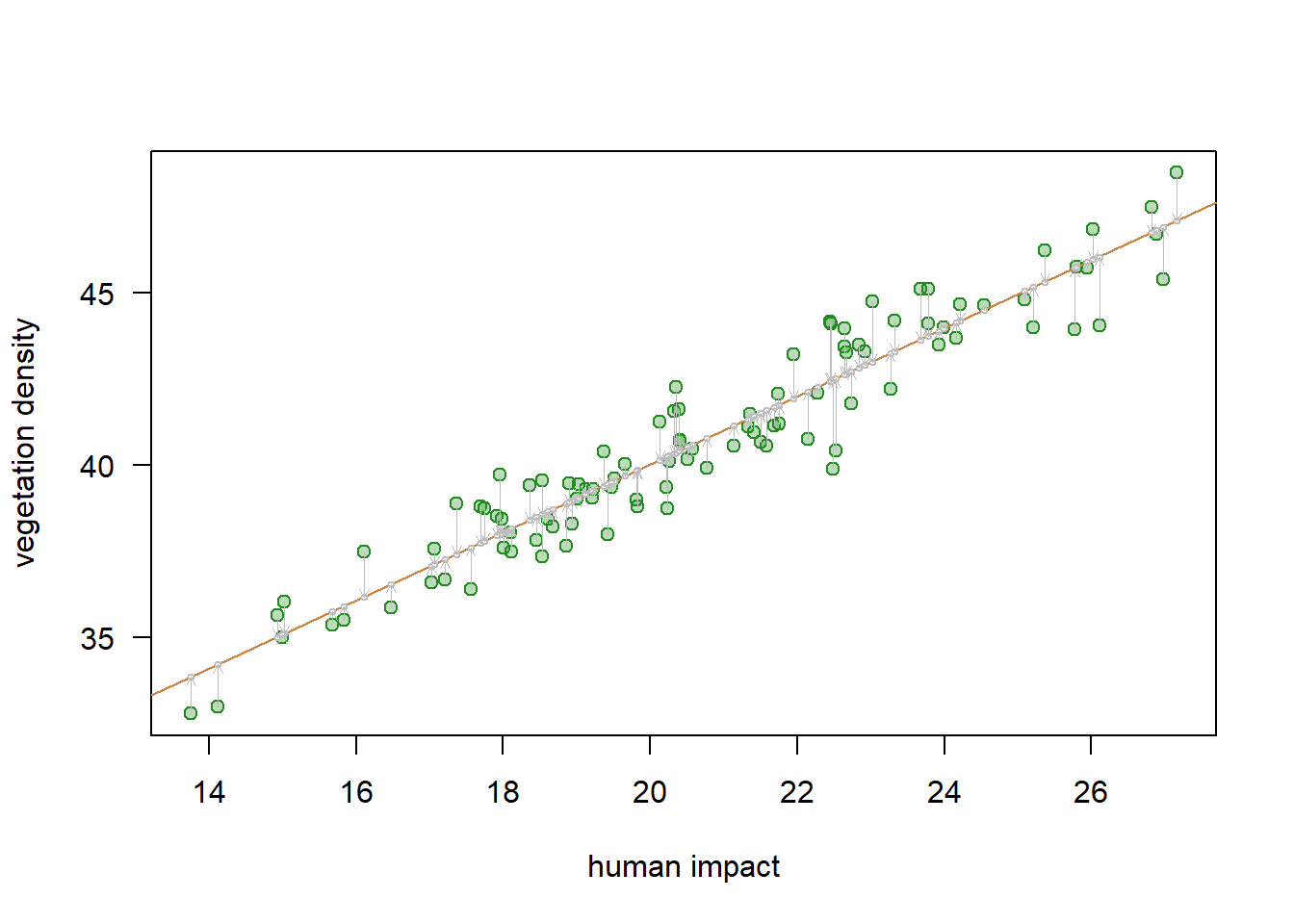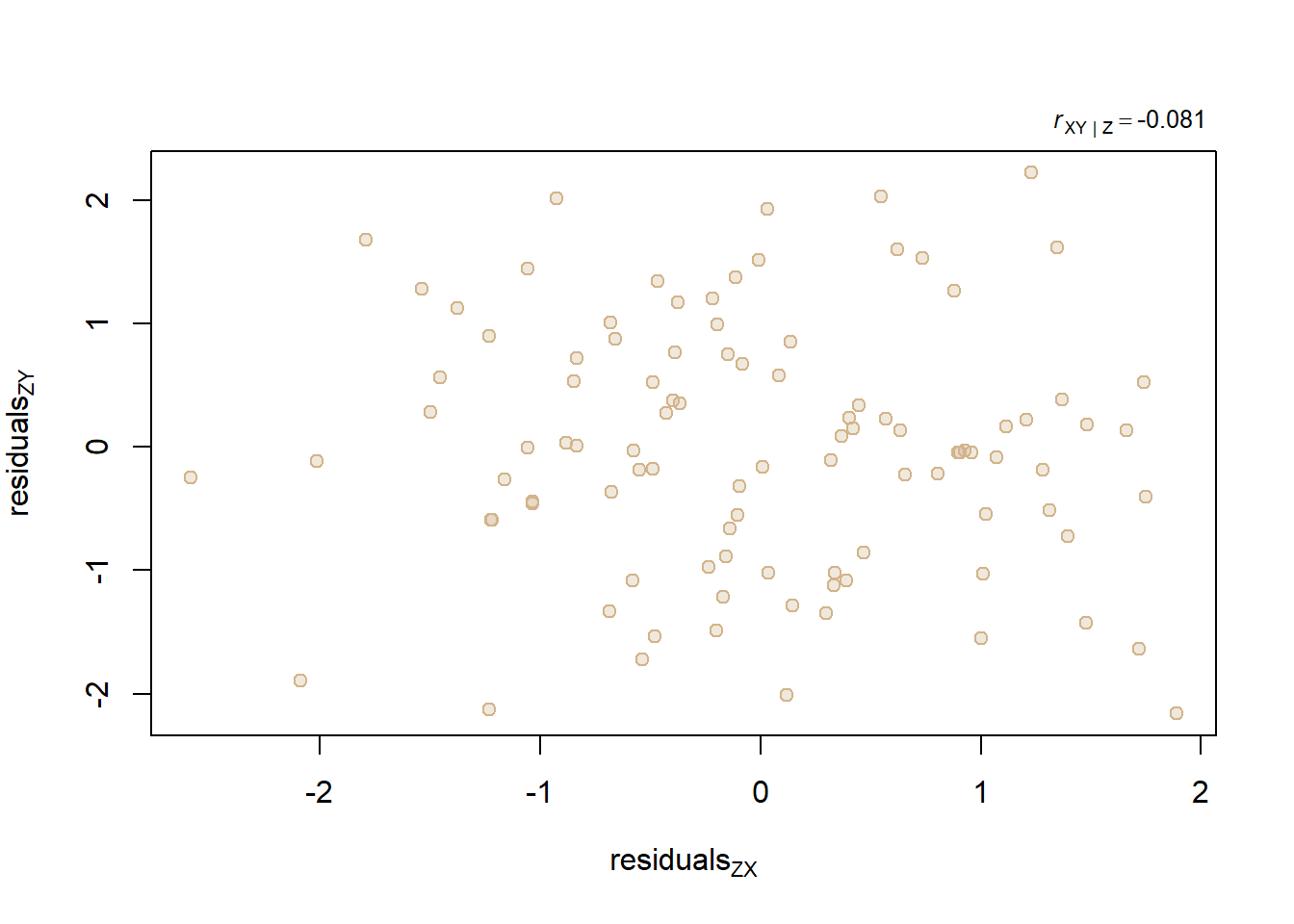## Partial Correlation

Partial correlation is an approach that evaluates correlation of variables x and y after accounting for possible influence of other variables. To explore this concept and illustrate analytical solutions in R, we will use a simple example involving three variables.

Please install and upload the library {ppcor} that we will use later for automated computation of partial correlation.

library(ppcor)     # upload library 'ppcor'
## Loading required package: MASS
options(digits=3)  # round all outputs to 3 significant figures 

We will first generate a dataset with three variables.

set.seed(1688)  # let's fix the set.seed value so the same results can be reproduced
human.impact <- rnorm(100, 20, 3)
data1 <- data.frame(human.impact,
vegetation.density=rnorm(100, 20, 1) + human.impact,
animal.diversity=rnorm(100, 20, 1) + human.impact)

Note that in this synthetic example, the variable ‘human impact’ strongly influences the other two variables (‘vegetation density’ and ‘animal diversity’). On the other hand, ‘vegetation density’ and ‘animal diversity’ are not interrelated directly and ‘human impacts’ are not causally controlled by either ‘vegetation density’ and ‘animal diversity’.

Let us first examine standard Pearson’s correlation coefficients

# NOTE: "cor" function applied to a matrix-like numerical object
#       will produce a correlation matrix
cor(data1)
##                    human.impact vegetation.density animal.diversity
## human.impact              1.000              0.954            0.952
## vegetation.density        0.954              1.000            0.901
## animal.diversity          0.952              0.901            1.000
# We can also carry out statistical testing using function "cor.test".
# Statements below illustrate that we can selectively access p values
# (or other values in function outputs) by accessing a specific list
# included in the output. In its default form, cor.test will
# report p values for the  null hypothesis "correlation coefficient = 0".
cor.test(data1$human.impact, data1$vegetation.density)$p.value ##  3.35e-53 cor.test(data1$human.impact, data1$animal.diversity)$p.value
##  3.1e-52
cor.test(data1$vegetation.density, data1$animal.diversity)$p.value ##  2.38e-37 The outputs above show that all variables are highly correlated, including strong correlation between ‘vegetation density’ and ‘animal diversity’. In all cases, p values are extremely small and unambiguously significant statistically. However, always keep in mind that even extremely small p values do not necessarily equate with strong correlation between the variables. In this case, the low p values indicate that r values are unlikely to be 0, but you should never interpret any statistical results solely on the basis of p values. # let's plot scatter plots for all pairs of variables (3 plots) # Note: you can use 'pairs' function to quickly plot all possible # pairs of variables, but the example below illustrates # how you can create a customized muti-panel plot on your own. mypar1 <- par(mfrow=c(1,3), mai=c(0.5,0.5,0.1,0.1), omi=c(0.1,0.1,0.1,0.1), pty='square') for (i in 1:3) { if(i == 1) ind <- c(1, 2) if(i == 2) ind <- c(1, 3) if(i == 3) ind <- c(2, 3) plot(data1[,ind], data1[,ind], xlab=colnames(data1)[ind], ylab=colnames(data1)[ind], pch=21, col='coral3', bg=adjustcolor('coral3', 0.3)) mtext(side=3, line=-1.5, adj=0.05, bquote(italic(r) == .(round(cor(data1[,ind], data1[,ind]),3))), cex=0.8) }par(mypar1) # reset graphic {par} options back to default setting Figure 1. Bivariate plots for the three variables used in this example. All pairs of variables are strongly correlated (Fig. 1), but in this synthetic example we know (as we wouldn’t have known in the case of real empirical data) that ‘animal diversity’ and ‘vegetation density’ are not directly related. This illustrates the fact that it may often be desirable to ‘remove’ the effect of a given variable (e.g., ‘human impact’) and measure correlation after the effect of that variable has been accounted for. This new (partial) correlation may provide a more realistic assessment of inter-relation between ‘vegetation density’ and ‘animal diversity’. ## Computing Partial Correlation For a simple case of three variables, we can compute xy|z (partial correlation of x and y given z) by regressing both x and y against z using a simple linear regression (OLS - ordinary least squares). For details on other more efficient ways for computing partial correlation and to learn more about different variants of partial correlations, please refer to Kim (2015), Commun Stat Appl Methods 22(6): 665-674. Available here: https://www.ncbi.nlm.nih.gov/pmc/articles/PMC4681537/ Note: OLS Residuals are deviations measured in the direction of the dependent variable y (i.e., differences between the observed and predicted value of y). This can be graphically visualized as follows (Fig. 2): reg.xy <- lm(data1$vegetation.density ~ data1$human.impact) plot(data1[,1], data1[,2], xlab='human impact', ylab='vegetation density', las=1, col='forestgreen', pch=21, bg=adjustcolor('forestgreen', 0.3)) abline(reg.xy, col='tan3') points(data1[,1], reg.xy$fitted.values, pch=21, bg='white', cex=0.5, col='gray')
arrows(data1[,1], data1[,2], data1[,1], reg.xy$fitted.values, lwd=0.5, col='gray', length=0.05)Figure 2. Visual illustration of residuals (arrows) for a simple linear (OLS) regression. We can now use residuals to compute partial (and semi-partial) correlations of ‘animal diversity’ and ‘vegetation density’ after minimizing the effect of the control variable ‘human impact’. Semi-partial correlations are computed by considering only a subset of interactions. For example, we can measure semi-partial correlation of ‘vegetation density’ and ‘animal diversity’ after removing the influence of ‘human impact’ on only one of the two evaluated variables. # first compute residuals for 'vegetation density' and 'animal diversity' res12 <- lm(data1$vegetation.density ~ data1$human.impact)$residuals
res13 <- lm(data1$animal.diversity ~ data1$human.impact)$residuals # now compute partial correlation (i.e., correlation of residuals) p.r <- cor(res12, res13) # you can also compute semi-partial correlations: #semi-partial cor.: 'veg.density' ('anim.div.' | 'h.impact') spr1 <- cor(data1$vegetation.density, res13)
#semi-partial cor.: 'anim.diver' ('veg.density' | 'h.impact')
spr2 <- cor(data1$animal.diversity, res12) rbind(partial.r_VD.AD=p.r, semipartial.r_VD=spr1, semipartial.r_AD=spr2) ## [,1] ## partial.r_VD.AD -0.0815 ## semipartial.r_VD -0.0244 ## semipartial.r_AD -0.0249 The partial correlation p.r = -0.081 is dramatically lower than the standard correlation. In fact, in this specific case, correlation is negative (although the value is so close to zero that the sign is, most likely, totally meaningless). Similar outcomes are produced when semi-partial correlations are computed. Note that whereas partial correlations estimate the association between pairs of variables after removing the effect of all other variables, semi-partial correlations estimate the associations between pairs of variables after removing the effect of a selected subset of other variables. It is also instructive to visualize the relationship between the X|Z and Y|Z residuals. plot(res12, res13, pch=21, col='tan', bg=adjustcolor('tan', 0.3), xlab=bquote(residuals[ZX]), ylab=bquote(residuals[ZY])) mtext(side=3, adj=0.99, line=.2, cex=0.8, bquote(italic(r)[XY][' | '][Z] == .(round(p.r,3))))Figure 3. A bivariate plot of residuals (an added-variable plot) for the two examined variables. The bivarate plot of those residuals (Fig. 3) suggests a shotgun pattern that typifies uncorrelated or poorly correlated variables. This differs dramatically from the original plot of the raw data. ## Testing for Statistical Significance of Partial Correlation The parametric partial correlation test is based on t statistics, but it differs subtly from the standard t test for significance of correlation. We can compute it as follows (Kim, 2015; and reference therein): t = partial.r * square root((n-2-g)/(1-partial.r^2)), where n is the total number of observations and g is the number of controlling variables (in our case just one: ‘human impact’). In our case, this can be translated into a set of R statements as follows: # let's compute t for partial correlation r t <- p.r*sqrt((nrow(data1)-2-1)/(1-p.r^2)) # corresponding two-tailed p-value with N-2-g degrees of freedom p <- 2*pt(-abs(t),nrow(data1)-3) # using standard t-test for correlation is not correct # and will yield incorrect t and p values # (although they may be close to correct values, as is the case here) wrong.way <- cor.test(res12, res13) cbind(correct=c(t=t, p=p), incorrect=c(t=wrong.way$statistic, p=wrong.way$p.value)) ## correct incorrect ## t -0.805 -0.809 ## p 0.423 0.420 Note that the correction becomes inconsequential when n is large and g is small. ## Automating partial correlations with the ppcor package The ppcor package, developed by Kim (2015), allows users to estimate both partial and semi-partial correlations for all variables of interest (e.g., xy|z, yz|x, x|yz, etc.). You can include more than three variables and use rank-based measures of association (i.e., Kendall tau and Spearman rho). # partial correlation (note: pearson is the default setting) # to compute partial rank correlations use method='kendall' or method='spearman' pcor(data1, method='pearson')  ##$estimate
##                    human.impact vegetation.density animal.diversity
## human.impact              1.000             0.7265           0.7113
## vegetation.density        0.727             1.0000          -0.0815
## animal.diversity          0.711            -0.0815           1.0000
##
## $p.value ## human.impact vegetation.density animal.diversity ## human.impact 0.00e+00 1.72e-17 1.59e-16 ## vegetation.density 1.72e-17 0.00e+00 4.23e-01 ## animal.diversity 1.59e-16 4.23e-01 0.00e+00 ## ##$statistic
##                    human.impact vegetation.density animal.diversity
## human.impact               0.00             10.413            9.966
## vegetation.density        10.41              0.000           -0.805
## animal.diversity           9.97             -0.805            0.000
##
## $n ##  100 ## ##$gp
##  1
##
## $method ##  "pearson" # semi-partial correlations (notice that this matrix is not symmetric) spcor(data1)  ##$estimate
##                    human.impact vegetation.density animal.diversity
## human.impact              1.000             0.2221           0.2126
## vegetation.density        0.315             1.0000          -0.0244
## animal.diversity          0.308            -0.0249           1.0000
##
## $p.value ## human.impact vegetation.density animal.diversity ## human.impact 0.0000 0.0271 0.0346 ## vegetation.density 0.0015 0.0000 0.8109 ## animal.diversity 0.0019 0.8067 0.0000 ## ##$statistic
##                    human.impact vegetation.density animal.diversity
## human.impact               0.00              2.244             2.14
## vegetation.density         3.27              0.000            -0.24
## animal.diversity           3.19             -0.245             0.00
##
## $n ##  100 ## ##$gp
##  1
##
## $method ##  "pearson" Note that the output (a list) incudes partial and semi-partial correlation coefficients (‘estimate’), p values (‘p.values’), and t statistics (‘statistic’). The values agree with our computation above even though {pcor} function uses a different algorithm. Note also that partial correlations of ‘human impact’ with the two other variables are still substantial and significant (as they should be given how we constructed our synthetic example). So partial correlation analysis removed secondary correlation imposed by ‘human impact’ variable and provided a drastically different assessement of the direct inter-relationship between ‘vegetation density’ and ‘animal diversity’. Let us consider other scenarios. 1. The case in which the ‘human impact’ variable does not influence the other two variables, but ‘vegetation density’ does in fact influence ‘animal diversity’. # let's fix the set.seed value so the same results can be reproduced set.seed(1044) human.impact <- rnorm(100, 20, 1) vegetation.density <- rnorm(100, 20, 1) data2 <- data.frame(human.impact, vegetation.density, animal.diversity = rnorm(100, 20, 1) + vegetation.density) # Pearson's correlation cor(data2) ## human.impact vegetation.density animal.diversity ## human.impact 1.0000 -0.0426 -0.0938 ## vegetation.density -0.0426 1.0000 0.6783 ## animal.diversity -0.0938 0.6783 1.0000 # Partial correlation # output limited to the first list only (partial r matrix) pcor(data2)  ##$estimate
##                    human.impact vegetation.density animal.diversity
## human.impact             1.0000             0.0287          -0.0884
## vegetation.density       0.0287             1.0000           0.6779
## animal.diversity        -0.0884             0.6779           1.0000

Note that partial correlation of ‘vegetation density’ and ‘animal diversity’ is comparable to the actual correlation between those two variables, as should be the case here.

2. The case in which (1) the ‘human impact’ variable influences ‘vegetation density’, but does not directly affect ‘animal diversity’; and (2) ‘vegetation density’ does influence ‘animal diversity’.

# let's fix the set.seed value so the same results can be reproduced
set.seed(1044)
human.impact <- rnorm(100, 20, 1)
vegetation.density <- rnorm(100, 20, 1) + human.impact
data3 <- data.frame(human.impact, vegetation.density,
animal.diversity = rnorm(100, 20, 1) + vegetation.density)
# Pearson's correlation
cor(data3)
##                    human.impact vegetation.density animal.diversity
## human.impact              1.000              0.711            0.551
## vegetation.density        0.711              1.000            0.790
## animal.diversity          0.551              0.790            1.000
# Partial correlation
pcor(data3)   
## \$estimate
##                    human.impact vegetation.density animal.diversity
## human.impact              1.000              0.539           -0.024
## vegetation.density        0.539              1.000            0.678
## animal.diversity         -0.024              0.678            1.000

Note here that partial correlations correctly indicate that ‘human impact’ and ‘vegetation density’ (partial r = 0.538) AND also ‘vegetation density’ and ‘animal diversity’ (partial r = 0.678) are inter-related directly, whereas partial correlation of ‘human impacts’ and ‘animal diversity’ approximates 0 (-0.024) even though the standard correlation of the two latter variables was substantial: r = 0.551.

## Cautionary notes

Note that partial correlation may be inflated in cases when the controlling variables are in fact uncorrelated. For example, Foote (2000) cautioned as follows: “The use of partial correlations is not without its own risks, however. If the two correlations that one is attempting to factor out are nonexistent, so that their estimates represent noise distributed around zero, then it is possible for the correlation of interest to be inflated when the others are partialed out.”

More generally, interpretation of causalities in partial correlation analysis is as risky as it would be in the case of any standard correlation analysis. In the example above we used a synthetic example when we knew that Z influenced both X and Y and we also knew that X and Y were not causally related. In the case of real empirical data, however, there is always possibility that Z (and also X and Y) are influenced by other unknown factors that are the real drivers of the observed patterns. The “unknown unknows” always undermine any causalities we may be tempted to postulate.

## Paleontological examples of partial correlation analysis

Eder, W., Hohenegger, J., Briguglio, A., 2015, Depth-related morphoclines of megalospheric tests of Heterostegina depressa D’ORBIGNY: Biostratigraphic and paleobiological implications.Palaios 32:110-117. link

Foote, M., 2000, Origination and extinction components of taxonomic diversity: Paleozoic and post-Paleozoic dynamics. Paleobiology 26(4):578-605. link

## Acknowledge and cite packages

When publishing your analyses, please always remember to cite packages that you are using in your scripts.

citation('ppcor')
##
## To cite package 'ppcor' in publications use:
##
##   Seongho Kim (2015). ppcor: Partial and Semi-Partial (Part)
##   Correlation. R package version 1.1.
##   https://CRAN.R-project.org/package=ppcor
##
## A BibTeX entry for LaTeX users is
##
##   @Manual{,
##     title = {ppcor: Partial and Semi-Partial (Part) Correlation},
##     author = {Seongho Kim},
##     year = {2015},
##     note = {R package version 1.1},
##     url = {https://CRAN.R-project.org/package=ppcor},
##   }
##
## ATTENTION: This citation information has been auto-generated from
## the package DESCRIPTION file and may need manual editing, see
## 'help("citation")'.